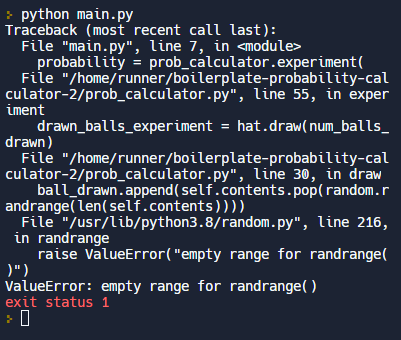# Probability Calculator Help on the Experiment part

Hi everyone,

So, I have been a bit stuck on the probability calculator as I cannot find a way to put back the balls. I have created an additional function called refill that is supposed to give back the same amount of balls.

I have been trying for the last few days to solve the issue and I almost solved it at one point.

I might be over complicating things so if you could give me some feedback that would be great.

Here is the code:

``````import copy
import random

from certifi import contents
# Consider using the modules imported above.

class Hat:

# When an object of type "Hat" is created, a set of variables with key arguments must be created, therefore **kwargs is appropriate to use
def __init__(self, **kwargs):
self.contents = list()

# We now want to obtain a list containing the number of balls of    different colours for which we use keys and values present in the dictionnary used
for key, values in kwargs.items():
step_up = 0
while step_up < values:
self.contents.append(key)
step_up += 1

self.copy_content = copy.deepcopy(self.contents)

# The balls passed in this function as arguments are to be withdrawn from the contents list
def draw(self, balls):
ball_drawn = list()
#index_ball_drawn = list()
step_up = 0
if balls > len(self.contents):
ball_drawn = self.contents
while step_up < balls:
ball_drawn.append(self.contents.pop(random.randrange(len(self.contents))))
#index_ball_drawn.append(random.randrange(len(self.contents)))
step_up += 1
#dic = {}
#for through in range(0,len(ball_drawn)):
#dic[ball_drawn[through]] = index_ball_drawn[through]
#dic = dict(zip(ball_drawn, index_ball_drawn))
return ball_drawn#, dic

def refill(self):
self.contents = self.copy_content

# Now, we want to create a function that give us the probability to draw certain amount and type of balls from the hat
def experiment(hat, expected_balls, num_balls_drawn, num_experiments):
won_games = 0
list_expected_balls = list()

for key, values in expected_balls.items():
step_up = 0
while step_up < values:
list_expected_balls.append(key)
step_up += 1

for go_through in range(num_experiments):
drawn_balls_experiment = hat.draw(num_balls_drawn)
for go_through in drawn_balls_experiment:
for through in list_expected_balls:
if go_through == through:
list_expected_balls.remove(go_through)
elif len(list_expected_balls) == 0:
won_games += 1
hat.refill()
return won_games/num_experiments
``````

At one point, I tried to create a dictionary with the balls drawn with their respective index to try to put them back exactly where they need to be put back.

I have tried using also a “try” and “except” and much more…

Any help is valuable at this point. I think I have just over complicated this.As you can see the refill function does not work otherwise I would not have gotten this error.

Thanks in advanceOne thing I noticed is when you create copy_contents you use deepcopy but when you do refill, you don’t.

I would not think it is necessary as I deepcopied its original value at the init() and then assigned that value in the refill function.

Why would there be a need to do a deepcopy once again?

I am just trying to understand here

Thanks for the tip.

Today, I finally managed to finish the challenge.

I know it ain’t optimal but at least it is doneThis topic was automatically closed 182 days after the last reply. New replies are no longer allowed.